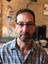Search
•Jack Orenstein

# Pipelines and Variables

Most interaction with marcel involves the execution of a pipeline. For example:

`ls -fr | select (f: now() - f.mtime < days(1))`

ls explores the current directory recursively (-r), returning only files (-f). The resulting File objects are piped to the select operator, which keeps only those that have changed in the past day.

You write a command like this, hit return, get your result. But you can also store a pipeline in a variable for later execution, much as you would when writing a function in any programming language.

To store the above command in a variable, use conventional assignment notation, putting the pipeline inside brackets:

`recent = [ls -fr | select (f: now() - f.mtime < days(1))`

You can run this command at any time by just using the variable as if it were an operator:

`recent`

You can also parameterize a pipeline. For example, the following pipeline explores the current directory recursively, and locates files with a given extension, (e.g. .py in util.py):

`ext = [e: ls -fr | select (f: f.suffix == e)]`

You can now run this pipeline, providing the extension of interest either as an argument:

`ext .py`

Or using flag notation, where the flag name is determined by the parameter's name:

`exp -e .py`